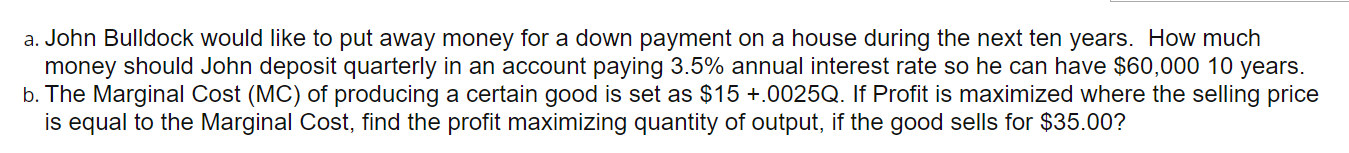# Question a. John Bulldock would like to put away money for a down payment on a house during the next ten years. How much money should John deposit quarterly in an account paying 3.5% annual interest rate so he can have $60,000 10 years. b. The Marginal Cost (MC) of producing a certain good is set as$15 +.0025Q. If Profit is maximized where the selling price is equal to the Marginal Cost, find the profit maximizing quantity of output, if the good sells for $35.00?T3IZ26 The Asker · CalculusTranscribed Image Text: a. John Bulldock would like to put away money for a down payment on a house during the next ten years. How much money should John deposit quarterly in an account paying 3.5% annual interest rate so he can have$60,000 10 years. b. The Marginal Cost (MC) of producing a certain good is set as $15 +.0025Q. If Profit is maximized where the selling price is equal to the Marginal Cost, find the profit maximizing quantity of output, if the good sells for$35.00?
More
Transcribed Image Text: a. John Bulldock would like to put away money for a down payment on a house during the next ten years. How much money should John deposit quarterly in an account paying 3.5% annual interest rate so he can have $60,000 10 years. b. The Marginal Cost (MC) of producing a certain good is set as$15 +.0025Q. If Profit is maximized where the selling price is equal to the Marginal Cost, find the profit maximizing quantity of output, if the good sells for \$35.00?